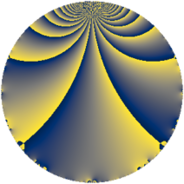# Properties

 Label 39.2.kLevel $39$ Weight $2$ Character orbit 39.k Rep. character $\chi_{39}(2,\cdot)$ Character field $\Q(\zeta_{12})$ Dimension $12$ Newform subspaces $2$ Sturm bound $9$ Trace bound $1$

# Related objects

## Defining parameters

 Level: $$N$$ $$=$$ $$39 = 3 \cdot 13$$ Weight: $$k$$ $$=$$ $$2$$ Character orbit: $$[\chi]$$ $$=$$ 39.k (of order $$12$$ and degree $$4$$) Character conductor: $$\operatorname{cond}(\chi)$$ $$=$$ $$39$$ Character field: $$\Q(\zeta_{12})$$ Newform subspaces: $$2$$ Sturm bound: $$9$$ Trace bound: $$1$$ Distinguishing $$T_p$$: $$2$$

## Dimensions

The following table gives the dimensions of various subspaces of $$M_{2}(39, [\chi])$$.

Total New Old
Modular forms 28 28 0
Cusp forms 12 12 0
Eisenstein series 16 16 0

## Trace form

 $$12 q - 2 q^{3} - 12 q^{4} - 2 q^{6} - 14 q^{7} - 2 q^{9} + O(q^{10})$$ $$12 q - 2 q^{3} - 12 q^{4} - 2 q^{6} - 14 q^{7} - 2 q^{9} + 12 q^{10} + 8 q^{13} - 14 q^{15} + 4 q^{16} + 4 q^{18} - 2 q^{19} + 22 q^{21} + 4 q^{22} + 18 q^{24} + 4 q^{27} + 18 q^{30} - 6 q^{31} + 16 q^{33} - 36 q^{34} - 36 q^{36} - 30 q^{37} - 38 q^{39} - 24 q^{40} - 16 q^{42} + 30 q^{43} - 20 q^{45} - 14 q^{48} + 18 q^{49} + 76 q^{52} + 46 q^{54} + 4 q^{55} + 28 q^{57} + 28 q^{58} + 44 q^{60} + 28 q^{61} + 16 q^{63} - 40 q^{66} - 8 q^{67} - 32 q^{70} + 12 q^{72} - 62 q^{73} - 18 q^{75} - 36 q^{76} - 80 q^{78} + 16 q^{79} - 14 q^{81} - 24 q^{82} - 8 q^{84} + 12 q^{85} - 34 q^{87} - 12 q^{88} + 10 q^{91} + 10 q^{93} + 64 q^{94} + 16 q^{96} - 18 q^{97} + 40 q^{99} + O(q^{100})$$

## Decomposition of $$S_{2}^{\mathrm{new}}(39, [\chi])$$ into newform subspaces

Label Dim $A$ Field CM Traces $q$-expansion
$a_{2}$ $a_{3}$ $a_{5}$ $a_{7}$
39.2.k.a $4$ $0.311$ $$\Q(\zeta_{12})$$ $$\Q(\sqrt{-3})$$ $$0$$ $$0$$ $$0$$ $$-10$$ $$q+(-\zeta_{12}-\zeta_{12}^{3})q^{3}+2\zeta_{12}q^{4}+(-2+\cdots)q^{7}+\cdots$$
39.2.k.b $8$ $0.311$ 8.0.56070144.2 None $$0$$ $$-2$$ $$0$$ $$-4$$ $$q+(-\beta _{1}-\beta _{2}+\beta _{3}-\beta _{4}+2\beta _{5}+\beta _{7})q^{2}+\cdots$$